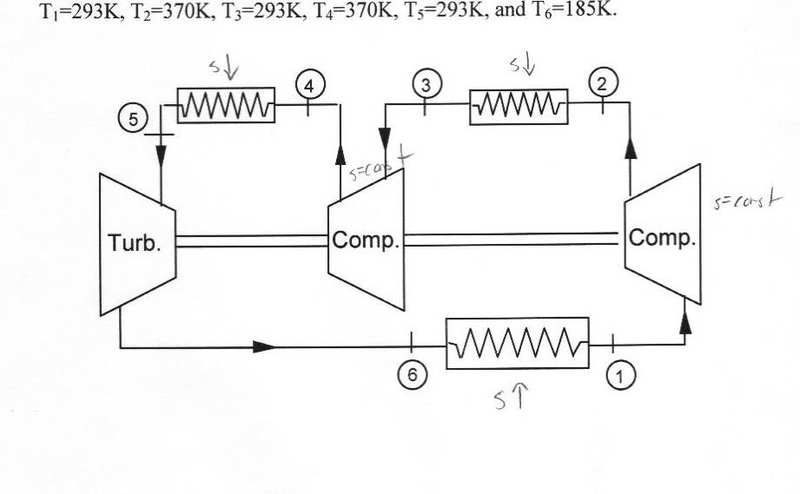# Refrigeration cycle with two compressors

#### murrdpirate0

1. The problem statement, all variables and given/known data

Here is the cycle:I am to assume cold-air standard applies.

I need to:
a)Draw a T-s diagram
b)Find where heat is transferred and work is done
c)Calculate the coefficient of performance

2. Relevant equations

COP = 1 / (TH/TL - 1)

3. The attempt at a solution

For the T-s diagram, as you can see on the image above, I just labeled what happens to entropy on each process (except I forgot to label the turbine, which like the compressor would be constant s ideally). I'm not really sure if this is an ideal case or not, so I guess s=const could be wrong.

Each compressor does work on the fluid, the fluid does work on the turbine, heat is transferred from the fluid to the surroundings in the intercoolers between the compressors, and heat is transferred from the surroundings to the fluid between 6 and 1. So that settles the second part of the hw.

Assuming that the fluid reaches equilibrium with the environment in the intercooling stage and the evaporation stage, TH would be 293K and TL would be 293. So COP would be infinity. Which obviously is impossible, but I think that could be the intention of this problem since we are starting to learn about irreversibility (and also because it seems to say that the turbine is driving the compressors, which would be a perpetual motion machine). But is it possible I'm neglecting something? Even if TH was higher, what would be the point of the second compressor? Like say T3=T5=TH=303. Then COP would calculate the same whether there was one compressor or two. Perhaps if there were only 1 compressor, T6 would be higher?

#### Q_Goest

Homework Helper
Gold Member
Hi murrdpirate0,
a) You're correct about dS = 0 for compressors and turbine assuming perfect isentropic performance (isentropic efficiency = 100%). And there is a change in entropy through the heat exchangers, but I'd suggest not showing this entropy change as an arrow. For the case of a fluid that's cooling at constant pressure, such as for the two heat exchangers where heat is rejected by the system, the entropy of the fluid drops, so showing an arrow pointing into the heat exchanger may be misleading. Or did you mean to show that entropy was dropping because the arrow is pointing down?

b) I think you've answered this one correctly.

c) It's been a long time since I did refrigeration cycles, but I believe TH is the high temperature reservoir and TL is the low temp reservoir. From Wikipedia:
where Thot and Tcold are the temperatures of the hot and cold heat reservoirs respectively.
Ref: http://en.wikipedia.org/wiki/Coefficient_of_performance
Read up on COP and try this part again.

### The Physics Forums Way

We Value Quality
• Topics based on mainstream science
• Proper English grammar and spelling
We Value Civility
• Positive and compassionate attitudes
• Patience while debating
We Value Productivity
• Disciplined to remain on-topic
• Recognition of own weaknesses
• Solo and co-op problem solving